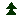Plantes nectarifères (N)
&
Plantes hôtes (H)

Annuelles & Bisannuelles

Arctotheca (N)
Brachycome (N)
Calendula officinalis (N)
Camassia (N)
Cleome spinosa (N & H)
Cosmos sulphureus (N)
Crinodendron (N)
Dipsacus fullonum (N)
Dolichos lablab (H)
Erysimum (N)
Giroflée jaune (N)
Godetia amoena (N)
Helianthus anuus (N)
Heliotropium (N)
Lunaria annua (N)
Lysimachia clethroides(N)
Nicotiana (N & H)
Phacelia tanacetifolia (N & H)
Petroselinum (H)
Petunia (N)
Silene vulgaris (N)
Tithonia rotundifolia
Tagetes erecta (N)
Tagetes patula (N)
Tropaelum (N & H)
Zinnia elegans (N)

Arbustes & Arbrisseaux

(Il manque les arbres hôtes)

Acacia (N) (H)
Angophora (N) (H)

Bauhinia galpinii (N) (H)
Brachychiton (N) (H)
Buddleja (N)
Callistemon (N)
Cestrum
Choisya ternata (N)
Cistus (N)
Ceanothus tomentosus (N)
Clerodendrum speciosissimum (N)
Cotoneaster (N)
Dorycnium hirsutum (N)
Dorycnium suffruticosum (N)
Eremophila (N)
Eriostemon myoporoide (N)
Hamelia patens (N)
Hardenbergia (N)
Hebe (N)
Heptacodium (N)
Hibiscus ( N)
Hymenolepis parviflora (N)
Hyssopus officinalis (N)
Lantana (N)
Lavandula (N)
Lavatera arborea (N)
Leonotis spp. (N)
Lonicera tatarica(N)
Medicago (N & H)
Pentas (N)
Philotheca myoporoides (N)
Physocarpus opulifolius (N)
Plectranthus ambiguus (N)
Tecoma capensis (N)
Rondeletia odorata (N)
Ruellia brittoniana (N)
Sumac (N)
Syringa (N)
Troene (N)
Tropaelum (N & H)
Ulmus (H)
Véronique arbustive (N)
Viburnum (N)
Zauschneria (N)

Bulbeuses & Rhizomateuses

Ail (N)
Anigozanthos (N) (H)
Paramongaia weberbaueri (N)
Dahlia (N)
Pancratium maritimum (N) & (H)
Saponaria officinalis (N)

Grimpantes

Araujia sericifera (N)
Aristolochia ringens (N)
Capucine (N & H)
Clerodendrum thomsoniae (N)
Duranta repens (N)
Hardenbergia violacea
Holboellia (N)
Ipomoea quamoclit (N)
Ipomoea tricolor (manquante) (N)
Jasminum (N)
Muehlenbeckia (N)
Lathyrus latifolius (N)
Petrea volubilis (N)
Quamoclit coccinea (N)
Thunbergia alata

Vivaces

Achillea (N)
Agastache foeniculum (N)
Ajania pacifica (N)
Ajuga (N)
Anthemis (N)
Anthyllis (H)
Arctotheca (N)
Aster (N)
Asclepias (N & H)
Aubrieta (N)
Centaurea (N & H)
Centranthus (N & H)
Coreopsis (N)
Cosmos bipinnatus (N)
Cymbalaria muralis (H)
Dianthus (N)
Digitalis (N)
Echinacea purpurea (N)
Echinops ritro (N)
Echium candicans (N)
Eryngium planum (N & H)
Eupatoriums (N)
Foeniculum vulgaire (H)
Gaura lindheimeri (N)
Gentiana acaulis (H)
Gentiana verna (H)
Giroflée quarantaine (N)
Gomphrena globosa (N)
Hesperis matronalis (N)
Huernia schneideriana (H)
Iberis (N)
Julienne des Dames
Knautia (N) & (H)
Leucanthemella serotina (N)
Liatris spicata (N)
Lobularia maritima
( ex Alyssum maritimum) (N)
Lupinus (N)
Marguerite (N)
Matthiola indica (N)
Mentha spicata (N)
Mentha (N & H)
Mirabilis jalapa
Muehlenbeckia (N)
Nepeta (N) & (H)
Ochna (N)
Oeillet (N)
Oenothera (N)
Origanum vulgare (N) & (H)
Pentas lanceolata (manquante) (N)
Phlox (N)
Plantago (N)
Primula (N)
Ratibida pinnata (N) & (H)
Rudbeckia (N)
Salvia (N)
Scabiosa caucasica (N) & (H)
Sedum spectabile (N & H)
Solidago (N)
Stachys lanata (N)
Stevia (N)
Tagetes lemonii (N)
Thymus (N & H)
Trachelium coeruleum (N)
Urtica (H)
Verbena bonariensis (N)
Verveine (N)
Viola (H)
Zauschneria californica (N)

Plantes mellifères